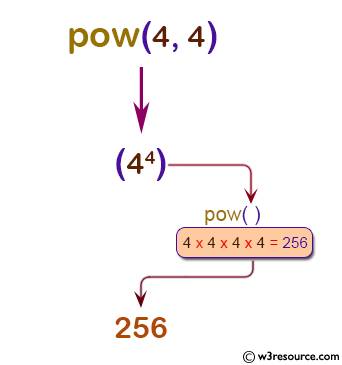# Python: pow() function

## pow() function

The pow() function is used to get the value of x to the power of y (xy). If z is present, return x to the power y, modulo z (computed more efficiently than pow(x, y) % z).

Version:

(Python 3.2.5)

Syntax:

```pow(x, y[, z])
```

Parameter:

Name Description Required /
Optional
x A number (the base) which is to be powered. Required
y A number (the exponent) which is to be powered with x. Required
z A number which is to be used for modulus operation. Optional

Example: Python pow() function

``````# positive a, positive b (a**b)
print(pow(4, 4))

# negative a, positive b
print(pow(-4, 4))

# positive a, negative b (a**-b)
print(pow(4, -4))

# negative a, negative b
print(pow(-4, -4))
``````

Output:

```256
256
0.00390625
0.00390625
```

Pictorial Presentation:Example: Python pow() with three arguments (x**y) % z

``````a = 3
b = 5
c = 7
print(pow(a, b, c))
``````

Output:

```5
```

Python Code Editor:

Previous: ord()
Next: print()

Test your Python skills with w3resource's quiz

﻿

## Python: Tips of the Day

How do I check if a list is empty?

For example, if passed the following:

```a = []
if not a:
print("List is empty")
```

Ref: https://bit.ly/2A4JXx9